# Short Notes: Solid State – Part 1# Crystalline and Amorphous Solids

Depending on the nature of order in the arrangement of the constituent particles (atom, molecules or ions), there may be two types of solids—crystalline solids and Amorphous solids.

#### (i) Crystalline Solids :

Here the constituent particles have long range order which means there is a regular pattern of arrangement of particles which repeats itself over long distances. They possess sharp melting point and undergo clean cleavage (cut). They show anisotropic behaviour.

Examples : NaCl, quartz, most of the metallic and non-metallic elements and their compounds.

#### (ii) Amorphous Solids :

(Greek word amorphos = shapeless) Here, the constituent particles do not possess long range order of arrangement but a short range arrangement and therefore they undergo irregular cut. They are pseudo solids and do not possess sharp melting points. They soften over a range of temperature and can be moulded and blown into various shapes. On heating they become crystalline at some temperature. They show isotropic behaviour.

Examples : Glass. Rubber and plastics. Glass is an example of supercooled liquid.

Amorphous solids are very useful materials. Glass, rubber and plastics find many applications in our everyday lives. Amorphous silicon being one of the best photovoltaic material used for conversion for sunlight into electricity.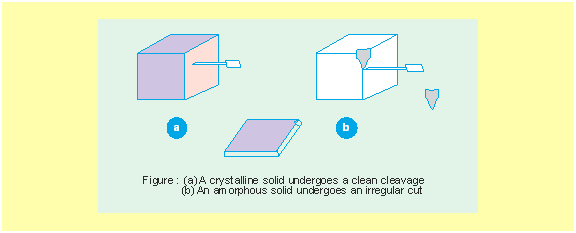# Classification of Crystalline Solids

Depending on the nature of intermolecular forces operating in the solids, they can be classified as follows:

 Types of Solid Constituent Particles Bonding/ Attractive Forces Examples Physical Nature Electrical Conductivity Melting Point 1. Molecular solids (i) Non polar Molecules Dispersion or London forces Ar, CCl4, H2, I2, CO2 Soft Insulator Very low (ii) Polar Dipole-dipole interactions HCL, SO2 Soft Insulator Low (iii) Hydrogen bonded Hydrogen bonding H2O(ice) Hard Insulator Low 2. Ionic Solids Ions Coulombic or electrostatic NaCl, MgO, ZnS, CaF2 Hard but brittle Insulators in solid state but conductors in molten state and in aqueous solutions High 3. Metallic solids Positive ions in a sea of delocalised electrons Metallic bonding Fe, Cu, Ag, Mg Hard but malleable and ductile Conductors in solid state as well as in molten state Fairly High 4. Covalent or network solids Atoms Covalent bonding SiO2(quartz), SiC, C(diamond), AIN Hard Insulators Very High C (graphite) Soft Conductor (exception)

# Space Lattice and Unit Cell

Space lattice or crystal lattice is a regular repeating arrangement of the constituent particles (atoms, ions or molecules of a crystal in a three dimensional space. A unit cell is a small portion of the lattice which produces the complete space lattice when repeated in all the three planes of space.Here, the points (called lattice points) represent the constituent particles in the three dimensional space lattice. The shaded portion denote a 3-d unit cell of space lattice.

Since, a unit cell is the smallest three dimensional unit of a space lattice, it possesses all the structural properties of a given lattice. A unit cell is our interest of study because it helps us to understand the structural properties of a crystal.

The unit cell for each lattice may be described by specifying the lengths of its sides (a, b and c) along X, Y and Z axes respectively and the angles α, β and γof which these axes intersect one another.

Based on the positions of particles in the unit cells, there can be two categories of unit cells:

#### (A) Primitive Unit Cells :

When the constituent particles are present only at the corners of the unit cells, these are called primitive unit cells or simple unit cells. There are seven types of primitive unit cells as described in the following table :

Seven Primitive Unit Cells and their Possible
Lattice types as Centred Unit Cells

 Crystal system Possible Lattice types Axial distances or edge lengths Axial angles Examples Cubic Primitive, Body-centred, Face-centred a = b = c α = β = γ = 90° NaCl, Zinc blende, Cu, KCl, Diamond, Alums Tetragonal Primitive, Body-centred a = b ≠ c α = β = γ = 90° White tin(Sn), SnO2, TiO2, CaSO4 Orthorhombic or Rhombic Primitive, Body-centred, Face-centred, End-centred a ≠ b ≠ c α = β = γ = 90° Rhombic sulphur, KNO3, BaSO4, PbCO3, CaCO3, K2SO4 Hexagonal Primitive a = b ≠ c α = β = 90° γ = 120° Graphite, ZnO, HgS(cinnabar), Cd, Mg, Zn, PbI2, Cd, ICl Rhombohedral or Trigonal Primitive a = b = c α = β = γ ≠ 90° Calcite(CaCO3), HgS(cinnabar), ICl, As, Sb, Bi, NaNO3 Monoclinic Primitive, End-centred a ≠ b ≠ c α  = γ = 90° β ≠90° Monoclinic sulphur, PbCrO4 Triclinic Primitive a ≠ b ≠ c α ≠ β ≠ γ ≠ 90° Na2SO4.10H2O.K2Cr2O7, CuSO4.5H2O, H3BO3#### (B) Non-primitive or Centred Unit Cells :

When the constituent particles are present not only at the corners but also at some other positions of the unit cells.

Again, non-primitive unit cells are of three types :

(i) Face-centred : When the particles are present at the centre of each face of the unit cell besides the corners.

(ii) End-centred : When particles are present at the centres of any two opposite faces in addition to the particles at the corners.

(iii) Body-centred : When in addition to the particles at the corners, there is one particle present at the centre within the body of the unit cell.

All the four types of unit cells i.e. Simple, face-centred, end-centred and body-centred are not found in every crystal system. Hence, there are only 14 types of space lattices (as described in above table) corresponding to the seven crystal systems. These fourteen types of space lattices are known as Bravais lattices, described as follows: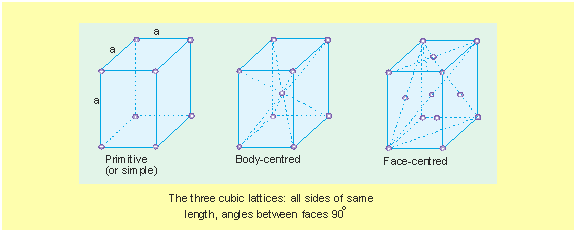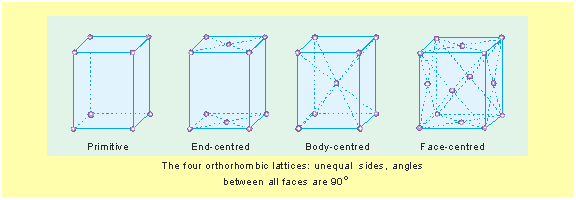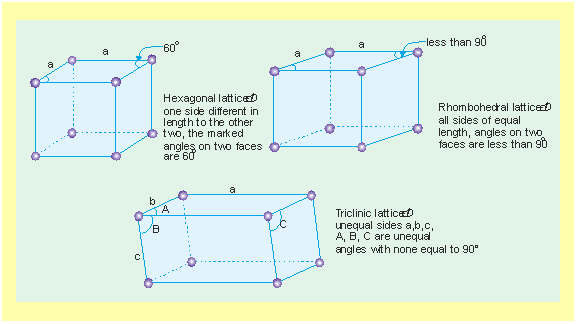# Calculation of number of atoms per unit cell

We know that, in a cube, there are 8 corners, 12 edges and 6 faces. Following diagrams show the contribution of atoms at the corners, face-centre and body-centre towards a unit cell of a cubic crystal systems: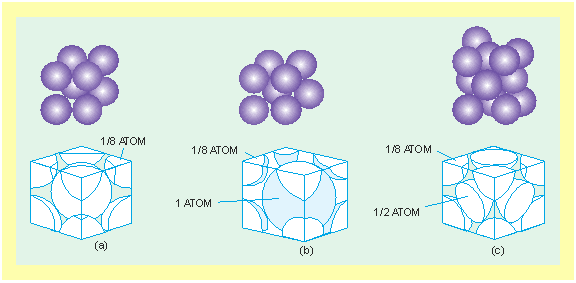From the above diagrams, it is clear that# Density of Lattice Matter# Coordination Number

A unit cell usually contains only a small number of atoms or ions or molecules (1 to 12).

In general, for any crystal system the number of atoms touching a particular atom is called its coordination number. Coordination number depends on the structure of a unit cell of crystal.

(i) For simple cubic structure (SC),

Coordination number = 6

(ii) For Face-centred cubic structure (FCC)

Coordination number = 12

(iii) For Body-centred cubic structure (BCC)

Coordination number = 8

In ionic crystal, coordination number may be defined as the number of oppositely charged ions surrounding a particular ion.

In ionic solids, the ratio of cation to anion is called the radius ratio. The radius ratio for a given coordination number is fixed. For example:

For Coordination number 4, radius ratio is (0.225 to 0.414)

And for Coordination number 6, Radius ratio is (0.414 to 0.732)

Further, for Coordination number 8, radius ratio is (0.732 to 1)

# Close packing of Constituent Particulars

#### (a) Close packing in one dimension

There is only one way of arranging spheres (i.e. atoms) in one dimensional close packed structure, that is to arrange them in a row and touching each other. In one dimensional close packed arrangement, the coordination number is 2.

#### (b) Close packing in two dimensions

(i) Square close packing : Two dimensional close packed structure can be generated by stacking (placing) the rows of close packed spheres. Two different ways for the same are shown in figures (a) and (b).(ii) Hexagonal close packing :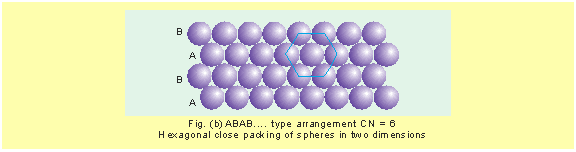#### (c) Close packing in three dimensions

##### (i) Hexagonal close packing (hcp) :

The first layer is formed utilizing maximum space, thus minimum space is left empty. In every second row, the particles occupy the depressions (or voids) between the spheres of the first row (see fig. below). In the third row, there is a vertical alignment of particles with those in the first row giving AB AB AB…… arrangement.

This structure has hexagonal symmetry and is known as hexagonal close packing (hcp) structure. This packing is more efficient and leaves small space which is unoccupied by spheres. In two dimension central sphere is in contact with six other spheres and only 26% space is free. In three dimensions, the coordination number is 12. A single unit cell has 4 atoms.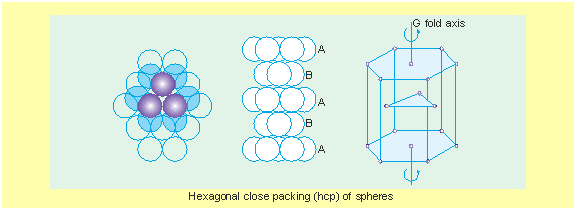##### (ii) Cubic close packing (ccp) :

Here also, if we start with hexagonal layer of spheres and second layer of spheres is arranged by placing the spheres over the voids of the first layer, half of these holes can be filled by these spheres. Suppose that spheres in the third layer are arranged to cover octahedral holes.

This arrangement leaves third layer not resembling with either first or second layer, but fourth layer is similar to first, fifth layer to second, sixth to third and so on giving pattern ABC ABC ABC….. This arrangement has cubic symmetry and is known as cubic-closed packed (ccp) arrangement. This is also called face-centred cubic (fcc) arrangement [Fig. (a) & (b)].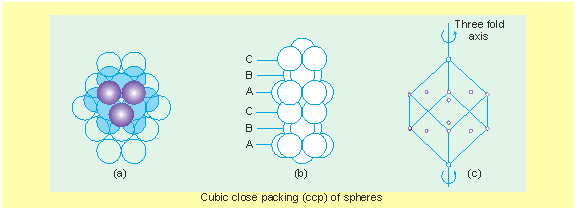The free space available in this packing is 26% and coordination number is 12.

##### (iii) Body centred close packing (bcp) :

The central sphere is at body centre and it is surrounded by 8 spheres at the corner of the cube. The coordination number of central sphere is 8. The efficiency of this type of packing is less, only 68% space is occupied and 32% remains free. A single unit cell has 2 atoms.